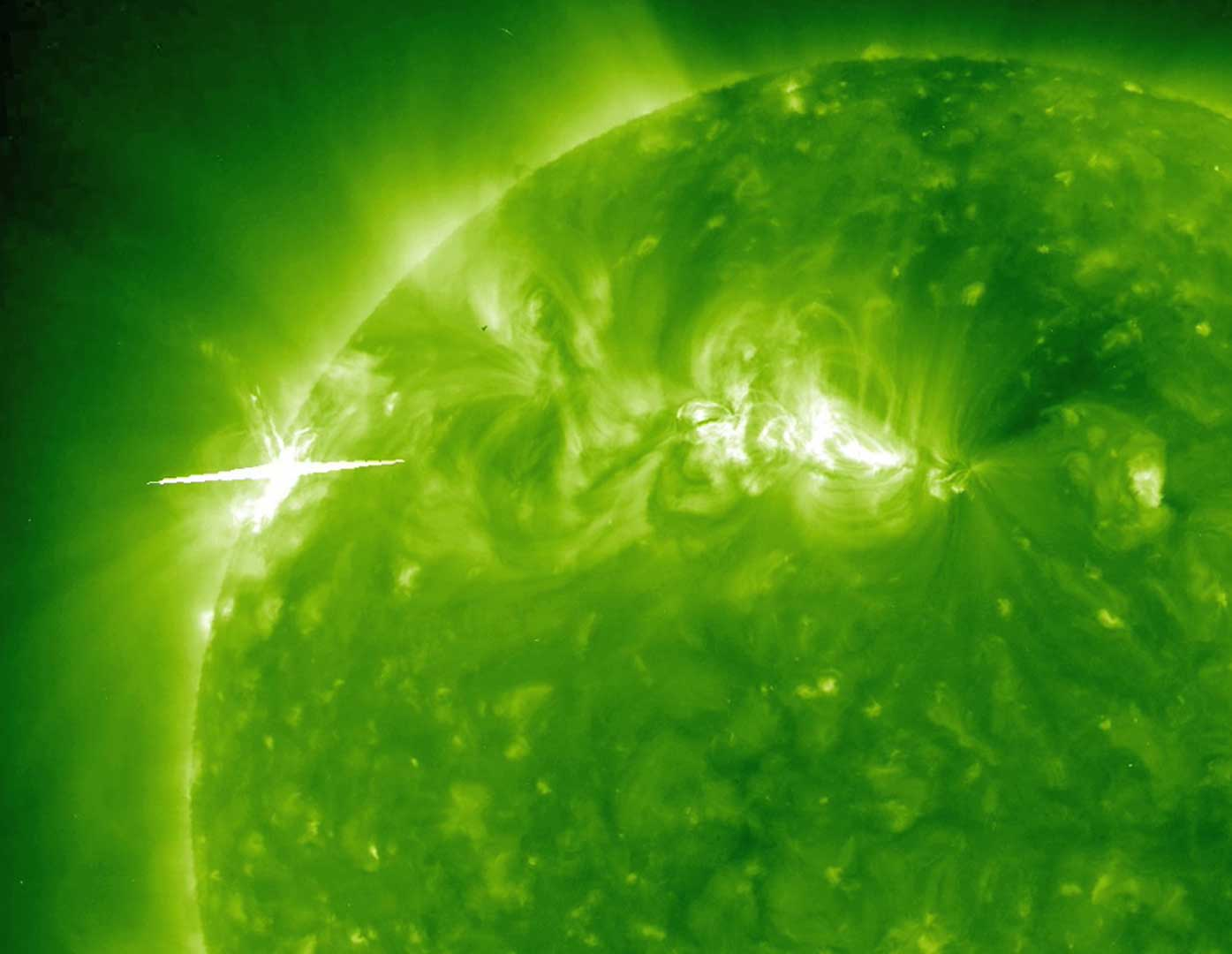µ IB | Energy Transfer

10 quick questions

10 minutes maximum! (can you do it in 5?)

1. Which one of the following methods of thermal energy transfer is most effective through solids?

• A. Conduction.
• B. Convection.
• D. Evaporation.

2. Which of the following is the best definition of the 'albedo' of a body?

• A. The fraction of radiation scattered compared to the incident radiation.
• B. The fraction of radiation absorbed compared to the incident radiation.
• C. The fraction of radiation reflected compared to a black body.
• D. The fraction of radiation rabsorbed compared to a black body.

3. What is the accepted average value of the Earth's albedo?

• A. 0.3
• B. 0.4
• C. 0.6
• D. 0.74. The 'solar constant' is a key value for considering global warming and the greenhouse effect. The value for Earth is taken to be about 1.4 kW m-2.

Which of these best describes the solar constant?

• A. The intensity of radiation from the Sun reflected by the Earth's atmosphere.
• B. The intensity of radiation from the Sun reflected by the Earth's surface.
• C. The intensity of radiation from the Sun incident on the Earth's atmosphere.
• D. The intensity of radiation from the Sun absorbed by the Earth's surface.

5-7. The graph below shows the how the intensity of radiation varies at different wavelengths for a given body.• A. The emission of radiation at different wavelengths from a perfect black body.
• B. The absorption of radiation at different wavelengths by the Earth.
• C. The transmission of radiation at different wavelengths through the Earth's atmosphere.
• D. The reflection of radiation at different wavelengths by the Earth's surface and atmosphere.

6. The radiation graph shown above is characteristic of a certain temperature. What happens to the height and position of the peak as the temperature falls?

Height of peak at lower temperature position of peak at lower temperature
A
Higher peak moves to the left
B
Higher peak moves to the right
C
Lower peak moves to the right
D
Lower peak moves to the left

7. The peak wavelength in the graph above is at a wavelength of 2.9 x 10-6 m. This corresponds to a temperature of....

• A. 10 3  0C
• B. 10-3  0C
• C. 10-3  K
• D. 10 3  K

8. A nearby star is twice the temperature of the Sun, but with only half the total surface area. What is the total power output of this star compared to the power output of the Sun (PSun)?

• A. 2 PSun
• B. 4 PSun
• C. 8 PSun
• D. 16 PSun9. Which of the following is a major greenhouse gas in the atmosphere?

• A. Water vapour
• B. Helium
• C. Nitrogen
• D. Oxygen

10. Carbon dioxide is a major greenhouse gas. Which of the following statements best describes the action of carbon dioxide in the atmosphere?

• A. It absorbs a wide spectrum of radiation from the Earth, and then re-radiates this in all directions.
• B. It absorbs a selective spectrum of radiation from the Earth, and then re-radiates this in all directions.
• C. It absorbs a wide spectrum of radiation from the Earth, and then re-radiates this back down to Earth.
• D. It absorbs a selective spectrum of radiation from the Earth, and then re-radiates this back down to Earth.